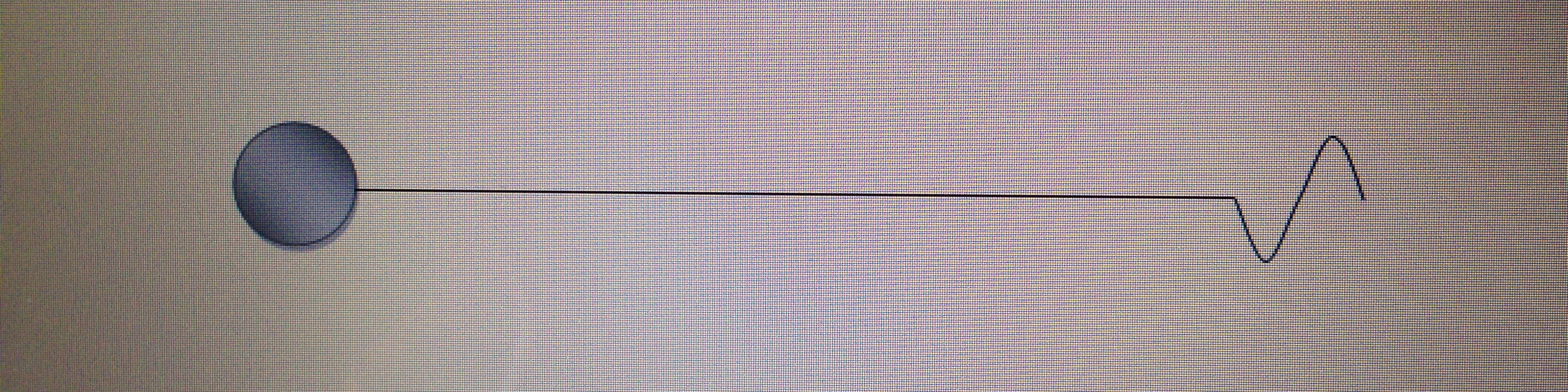## Wave motion- some basics

To understand what actually happens in wave motion and to realise where our great physicists went wrong, first we will have to know what a wave is. A wave is nothing but a pocket (or quantum) of energy in motion. As this energy pocket moves through a medium, its particles oscillate either up and down (transverse wave) or to and fro (longitudinal wave). Transverse and longitudinal waves are thus named to indicate the direction of oscillation of the medium’s particles with respect to the motion of the energy pocket.

As a wave moves ‘forward’, the energy quantum of the wave gets transmitted from one place to another. Whether it is a transverse wave (e.g. water wave) or a longitudinal wave (sound wave), what ultimately occurs is same i.e. energy transmission- But then why the medium’s particles oscillate transversely in some instances and to and fro at other times? Before we can dive into such depths and learn why there are two types of waves, we will have to unlearn some misconceptions that we may have allowed subconsciously into our minds since our school days.

### Frequency of a wave

We always tend to imagine a wave as a series of many ‘interconnected’ waves. This is one of the major misconceptions that stop us from understanding wave mechanics correctly. A wave is just a single pulse of energy. This need not be ‘flanked’ by a series of waves to be considered as a proper wave. Imagine that an electric sound vibrator produces the following sound wave on stimulation.We can see that the vibrator produces 15 waves per second or 1 wave each 0.04sec. But see what happens if the vibrator is switched on only for a brief period (0.04sec) each second –The source (vibrator) here releases only one wave or energy pocket per second. Does this mean that the resultant wave’s frequency is 1/sec? Of course not. Unlike what you may think, the frequency of the above ‘individual’ wave will remain same as that of the original uninterrupted ‘complete’ wave (i.e. 15 Hz). But how can the frequency of an ‘isolated wave’ is same as that of a ‘continuous wave’? To clarify this we should know how to calculate a wave’s frequency.

We all know that frequency indicates the number of cycles or waves crossing a reference point or number of waves received by a detector in unit time (which is usually 1 second). That doesn’t mean that we need to count the number of waves for one full second (or minute) to know the frequency of a wave. (If we were to, then what would be the resultant wave’s frequency if the above vibrator gets turned off for ever after releasing just one single pulse of energy or wave? Would it be 1/sec or 1/minute or 1/century?)

To know the frequency of any ‘individual’ wave, we have to note how long that wave takes to cross the point of interest or how long that wave is in ‘contact’ with the reference point while crossing the same. In other words this is the time period during which the wave transfers its energy quantum (e) to a receiver. I call this period as the impact time or energy transfer time (t) of that particular wave. (Some physicists call this as the period of a wave)Once we know the impact time of a wave, then calculating its frequency is easy. If one wave takes time ‘t’ to cross a point, how many identical waves will cross the point in 1 second? The answer is 1/t. So frequency is the inverse of impact time.

f = 1 / t

Impact time of a wave in turn depends upon its velocity and wavelength. We will discuss more about this later.

Having defined what is impact time, let’s now deduce the wave energy equation (i.e. how much energy a wave transmits to a target) while we are here.

### Energy transmitted by a wave (E)

Imagine that each wave or pulse carries energy quantum ‘e’ and delivers the same to a target over a period of time ‘t’.

We know that Energy (or capacity to do work) is always expressed per second. So if a wave transmits energy ‘e’ in time ‘t’, how much energy (E) does it transmit in one second?

Energy delivered in ‘t’ sec = e

So Energy delivered in 1 sec E = e / t

That is  E = e . 1 / t

We have seen above that frequency f = 1 / t

So rewriting the above equation gives us E = e f

(‘E’ is the energy transmitted by a wave in 1 second, ‘e’ the energy quantity carried in each pulse and ‘f’ is the frequency of the wave)

We can see that the above equation is similar to the energy equation of EM waves which is E=h ν   (‘h’ is the Planck’s constant and represents the energy quantum in each pulse of EM wave and ‘ν’ (nu) is the frequency of the EM wave)

Go to Next Page

Go to Previous Page

Go to Main Index